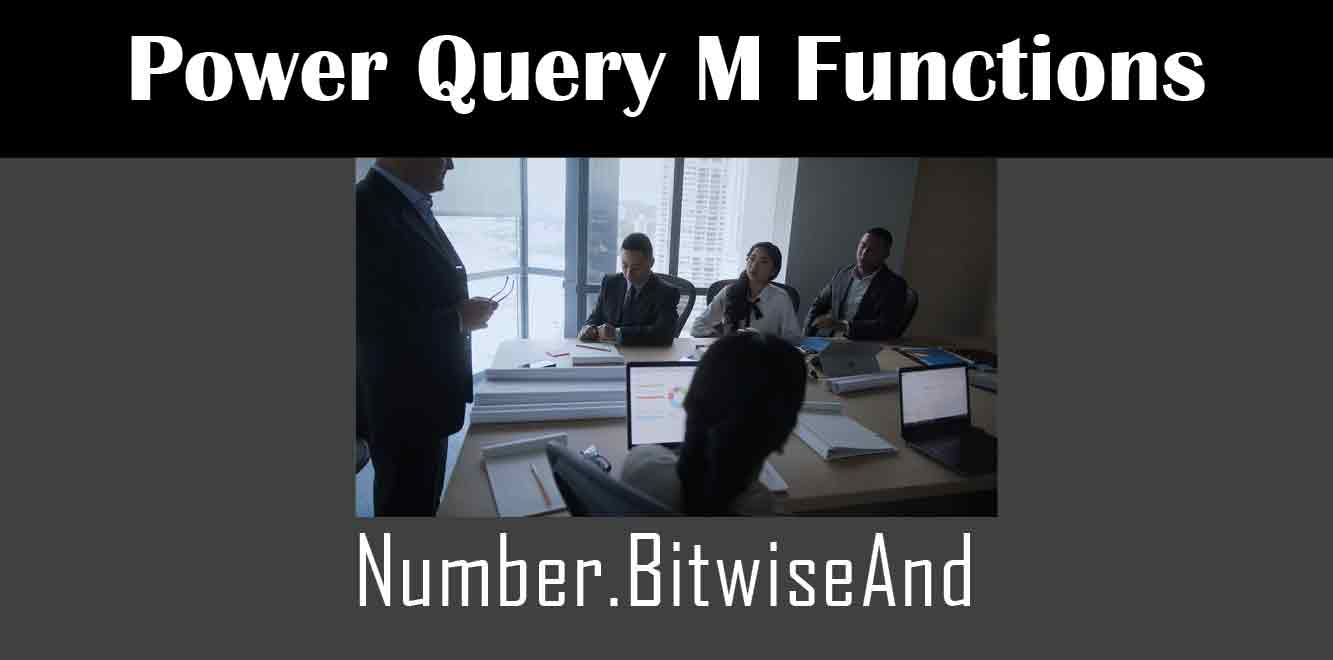# Number.BitwiseAnd

## ### What is Number.BitwiseAnd?

Before we dive into the M code behind Number.BitwiseAnd, let’s first understand what this function does. The Number.BitwiseAnd function is used to perform a bitwise AND operation on two numbers. In other words, it compares the binary representation of two numbers and only returns the bits that are set in both numbers.

For example, if we perform a bitwise AND operation on the numbers 5 and 3, we get the result 1. This is because the binary representation of 5 is 101 and the binary representation of 3 is 011. When we perform a bitwise AND operation on these two numbers, we get the binary representation 001, which is equivalent to the decimal number 1.

## The M Code Behind Number.BitwiseAnd

Now that we know what Number.BitwiseAnd does, let’s take a look at the M code behind this function. The M code for Number.BitwiseAnd is fairly simple, and it looks like this:

(Number as number, BitwiseValue as number) => Number band BitwiseValue

``` Let's break down this code to understand what it's doing. The first line defines the two parameters that the function takes: Number and BitwiseValue. Number is the number that we want to perform the bitwise AND operation on, and BitwiseValue is the value that we want to compare it to. The second line is where the actual bitwise AND operation is performed. The 'band' keyword is used to perform a bitwise AND operation on the two numbers, and the result is returned as the output of the function. How to Use Number.BitwiseAnd Now that we know how Number.BitwiseAnd works and what the M code behind it looks like, let's take a look at how we can use this function in Power Query. To use Number.BitwiseAnd in Power Query, we first need to create a new query. We can do this by clicking on the 'New Source' button in the Home tab of the Power Query Editor. Once we've created our new query, we can start to write our M code. To use Number.BitwiseAnd, we simply need to call the function and pass in the two numbers that we want to compare. For example, if we wanted to compare the numbers 5 and 3, we would write the following code: ```

Number.BitwiseAnd(5, 3)

``` This code would return the value 1, which is the bitwise AND of the numbers 5 and 3. In conclusion, the M code behind the Power Query M function Number.BitwiseAnd is a simple yet powerful function that can be used to perform bitwise AND operations on numbers. By understanding how this function works and how to use it in Power Query, you can leverage its power to transform and cleanse your data with ease. Power Query and M Training Courses by G Com Solutions (0800 998 9248)Power Query and M Intensive Training Course £1,050.00 – £26,550.00 Select optionsContinue Loading DonePower Query and M Introduction £395.00 – £9,750.00 Select optionsContinue Loading DonePower Query and M Intermediate £395.00 – £9,750.00 Select optionsContinue Loading DonePower Query and M Advanced £395.00 – £9,750.00 Select optionsContinue Loading Done Upcoming Courses Contact Us Subject Your Name (required) Company/Organisation Email (required) Telephone Training Course(s) Power BI Intensive TrainingPower BI introduction Power BI IntermediatePower BI AdvancedDAXPower Query MPower BI CertificationPower BI AdministrationPower PlatformPower AutomatePower AppsOTHER Your Message Upload Example Document(s) (Zip multiple files) ```
``` ```
``` ```
``` ```
``` ```
``` ```